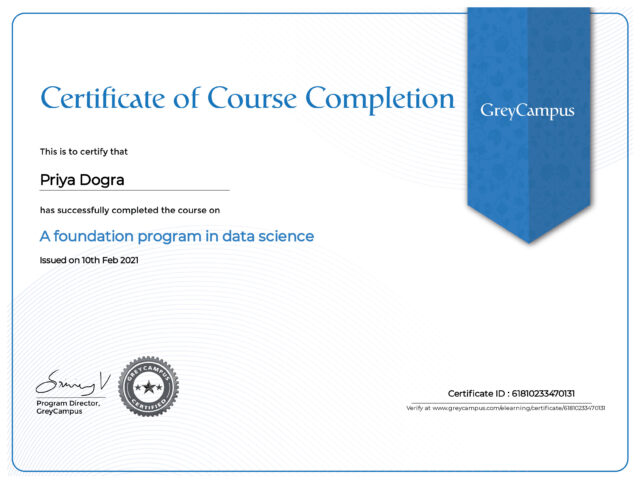Home Certification A Foundation Program in Data Science Quiz Answers – Grey Campus

# A Foundation Program in Data Science Quiz Answers – Grey Campus

0## Enroll Here: A Foundation Program in Data Science Certification

Question 1: Fill in the blanks with the correct option(s): Logistic regression is a ____________ regression technique that is used to model data having a ________ outcome

• linear, numeric
• linear, binary
• nonlinear, numeric
• nonlinear, binary

Question 2: Which of the following is NOT a supervised learning?

• PCA
• Decision Tree
• Linear Regression
• Naive Bayesian

Question 3: Which of the following is the method to find the best fit line for data in Linear Regression?

• Least Square Error
• Maximum Likelihood
• Logarithmic Loss
• Both A and B

Question 4: Which of the following assumption in regression modelling impacts the trade-off between under-fitting and over-fitting the most?

• The polynomial degree
• Whether we learn the weights by matrix inversion or gradient descent
• The use of a constant-term
• None of the above

Question 5: Which one of the following statements is true regarding residuals in regression analysis?

• Mean of residuals is always zero
• Mean of residuals is always less than zero
• Mean of residuals is always greater than zero
• There is no such rule for residuals.

Question 6: Q7. Which of the one is true about Heteroskedasticity?

• Linear Regression with varying error terms
• Linear Regression with constant error terms
• Linear Regression with zero error terms
• None of these

Question 7: Q10. To test linear relationship of y(dependent) and x(independent) continuous variables, which of the following plot best suited?

• Scatter plot
• Bar chart
• Histograms
• None of these

Question 8: Which of the following is true about “Ridge” or “Lasso” regression methods in case of feature selection?

• Ridge regression uses subset selection of features
• Lasso regression uses subset selection of features
• Both use subset selection of features
• None of above

Question 9: Which of the following options is true regarding “Regression” and “Correlation”? Note: y is the dependent variable and x is an independent variable.

• The relationship is symmetric between x and y in both.
• The relationship is not symmetric between x and y in both.
• The relationship is not symmetric between x and y in case of correlation but in case of regression it is symmetric.
• The relationship is symmetric between x and y in case of correlation but in case of regression it is not symmetric.

Question 10: Which of the following methods does not have a closed form solution for its coefficients?

• Ridge regression
• Lasso
• Both Ridge and Lasso
• None of both

Question 11: Which of the following step/assumption in regression modeling impacts the trade-off between under-fitting and over-fitting the most?

• The polynomial degree
• Whether we learn the weights by matrix inversion or gradient descent
• The use of a constant-term
• None of the above

Question 12 : Let’s say a “Linear regression” model perfectly fits the training data (train error is zero). Now, Which of the following statement is true?

• You will always have test error zero
• You can not have test error zero
• None of the above
• Both A and B

Question 13: Which of the following indicates a fairly strong relationship between X and Y?

• Correlation coefficient = 0.9
• The p-value for the null hypothesis Beta coefficient =0 is 0.0001
• The t-statistic for the null hypothesis Beta coefficient=0 is 30
• None of these

Question 14: Which of the following algorithm are not an example of an ensemble learning algorithm?

• Random Forest
• Extra Trees
• Decision Trees

Question 15: Which of the following is/are true while applying bagging to regression trees? 1.We build the N regression with N bootstrap sample. 2.We take the average the of N regression tree. 3. Each tree has a high variance with low bias.

• 1 and 2
• 2 and 3
• 1 and 3
• 1,2 and 3

Question 16: How to select best hyperparameters in tree based models?

• Measure performance over training data
• Measure performance over validation data
• Both of these
• None of these

Question 17: What are tree based classifiers?

• Classifiers which form a tree with each attribute at one level.
• Classifiers which perform series of condition checking with one attribute at a time.
• Both the options given above.
• None of the above

Question 18: How will you counter over-fitting in decision tree?

• By pruning the longer rules
• By creating new rules
• Both By pruning the longer rules’ and ‘ By creating new rules’
• None of the option

Question 19: Which of the following sentence(s) is/are correct?

• In pre-pruning a tree is ‘pruned’ by halting its construction early.
• A pruning set of class labeled tuples is used to estimate cost complexity.
• The best pruned tree is the one that minimizes the number of encoding bits.
• All of the above

Question 20: Which one of these is not a tree based learner?

• CART
• ID3
• Bayesian Classifier
• Random Forest